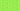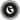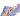PricingResources

# Variable cost: definition, formula and examplesWritten by

To accurately forecast corporate expenses, you need to learn how to calculate variable costs. But what are variable costs and how do they compare to fixed expenses? Find out more about what qualifies as ‘variable’ below.

## What is variable cost?

Business expenses are broken up into two main categories: fixed and variable. A variable cost is any business expense that changes according to production output. In other words, if your business produces more units, variable costs will also increase. They rise along with production increases and fall when there’s a decrease in production. For example, the amount your business spends on raw materials is variable, because it will fluctuate depending on your production needs.

In accounting, variable costs are looked at through a short-term lens because you can adjust them quickly by shifting production levels.

## Variable costs vs. fixed costs

To better understand the answer to ‘what is variable cost’, it’s helpful to compare it to the other type of expense – fixed costs. Fixed costs remain the same no matter how much (or little) you’re producing. Whether or not you make sales, you’ll still need to pay the same fixed costs. Examples of fixed costs include:

• Rent

• Office supplies

• Insurance

An employee’s salary would be considered a fixed cost, while sales commissions are variable. While fixed costs do change over a long-term period, this change isn’t related to production. For example, an employee might receive a raise in their salary after an annual review. However, this is unrelated to how many units were produced. At the same time, the employee might receive a sales commission directly tied to production, making it variable.

## How to calculate variable cost

To calculate fixed costs, you simply add them together to reach a total sum. By contrast, variable costs are calculated using multiplication. You can plug production data into the variable cost formula to determine total cost.

### Variable cost formula

• Total Variable Cost = Total Quantity of Products Produced x Variable Cost Per Unit

For example, if a pencil factory produced 10,000 boxes of pencils in the most recent accounting period, at a per-unit cost of £1.50, the total variable cost would be £15,000.

Of course, to use the variable cost formula you first need to calculate the variable per-unit cost. The formula for this is:

• Variable Cost Per Unit = Total Variable Costs / Total Units Produced

You simply divide your total variable costs from the accounting period in question by the total number of units produced.

## Variable cost examples

So, what types of costs qualify as variable? Anything directly related to variations in your business’s production activity falls under this definition. Here are a few variable cost examples:

• Raw materials: This is one of the first variable costs to be aware of, also called direct materials cost.

• Production supplies: The amount you spend on production supplies will be directly impacted by the volume of units you produce.

• Transaction fees: If your business accepts credit cards and needs to pay fees per transaction, for example, this qualifies as a variable cost.

• Piece rate labour: If you pay your workers for each unit that’s produced, piece rate labour would be included as a variable cost.

• Sales commissions: As mentioned above, sales staff are compensated according to the volume of products or services sold.

• Billable wages: Do you pay employees by the hour? If so, this could be a variable cost as employees will work more billable hours when needed. However, salaries qualify as a fixed cost.

## The bottom line

It’s important to look at variable vs. fixed costs, because if your variable costs are higher, this indicates that your business is turning a consistent profit. On the other hand, higher fixed costs in relation to variable costs indicate that profits are higher per-unit once the break-even point has been achieved. You’ll need to look at both figures together to get the full profitability picture.

## We can help

GoCardless helps you automate payment collection, cutting down on the amount of admin your team needs to deal with when chasing invoices. Find out how GoCardless can help you with ad hoc payments or recurring payments.Contact sales

Sales

Support